# If 2.09g of the vapor of a volatile liquid is able to fill a 498-mL flask...

If 2.09g of the vapor of a volatile liquid is able to fill a 498-mL flask at 370C and 617mm Hg, what is the molecular weight of the liquid?

PV = nRT

P = 0.812 atm

V = 0.498 L

T = 310K

0.812*0.498 = n*0.0821*310

0.404 = n * 25.5

n = 0.404/25.5 = 0.0158 mole

0.0158 mole = 2.09 g / molar mass

molarn mass = 2.09/0.0158 = 132.3 g. mol^-1

##### Add Answer to: If 2.09g of the vapor of a volatile liquid is able to fill a 498-mL flask...
Similar Homework Help Questions
• ### A volatile organic liquid with known molecular weight is added to a vapor density flask which has a known volume. The fl...

A volatile organic liquid with known molecular weight is added to a vapor density flask which has a known volume. The flask is heated using a boiling water bath until all of the liquid has evaporated. After heating for an additional 1 or 2 minutes, the flask is removed from the boiling water bath, corked, and cooled using a room temperature water bath. Predict the mass of condensed vapor. Temperature of the hot water bath (ºC): 99.4 Volume of the...

• ### 1. A volatile organic liquid with known molecular weight is added to a vapor density flask which has a known volume. The...

1. A volatile organic liquid with known molecular weight is added to a vapor density flask which has a known volume. The flask is heated using a boiling water bath until all of the liquid has evaporated. After heating for an additional 1 or 2 minutes, the flask is removed from the boiling water bath, corked, and cooled using a room temperature water bath. Predict the mass of condensed vapor. Temperature of the hot water bath (ºC): 99.5 Volume of...

• ### The pressure inside a sealed Erlenmeyer flask containing 5 mL of a volatile liquid was measured...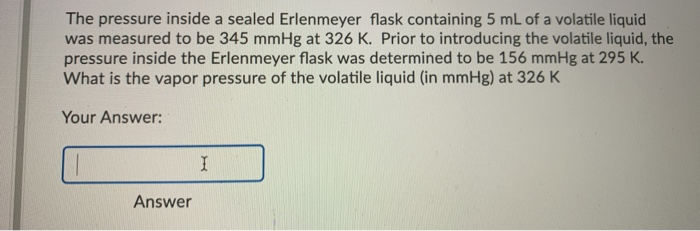The pressure inside a sealed Erlenmeyer flask containing 5 mL of a volatile liquid was measured to be 345 mmHg at 326 K. Prior to introducing the volatile liquid, the pressure inside the Erlenmeyer flask was determined to be 156 mmHg at 295 K. What is the vapor pressure of the volatile liquid (in mmHg) at 326 K Your Answer: I Answer

• ### THE MOLAR MASS OF A VOLATILE LIQUID 1. A foil-covered flask was found to have a...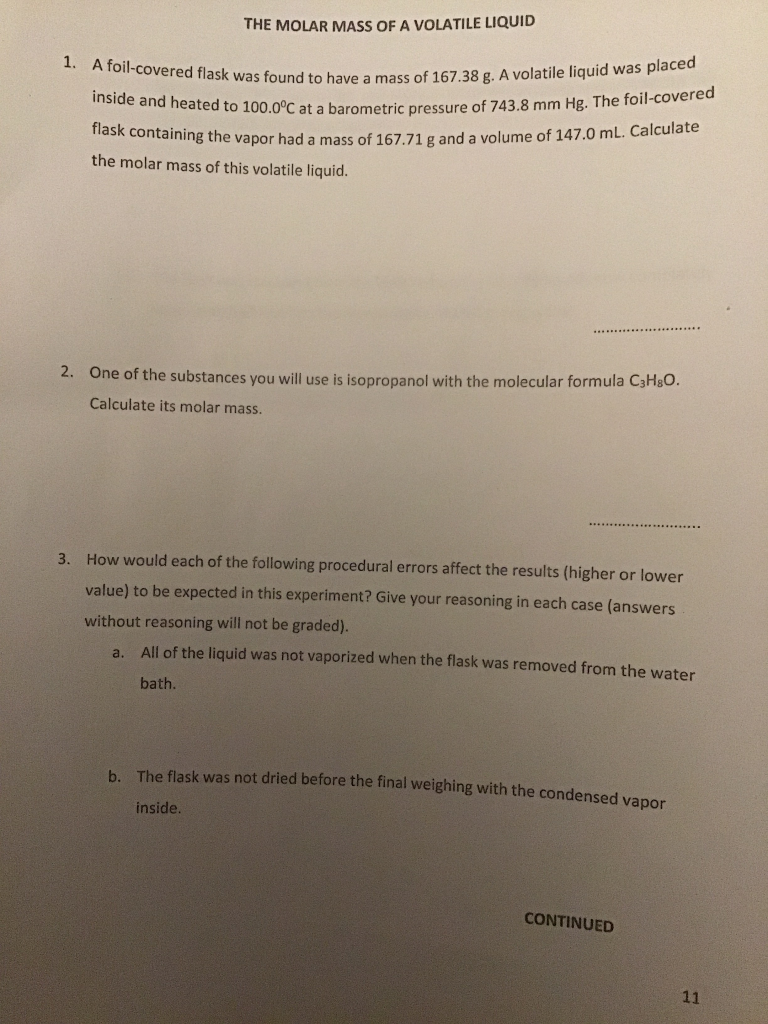THE MOLAR MASS OF A VOLATILE LIQUID 1. A foil-covered flask was found to have a mass of 161.38 have a mass of 167.38 g. A volatile liquid was placed L00.0°C at a barometric pressure of 743.8 mm Hg. The foil-covered inside and heated to 100.0°C at a barometric press flask containing the vapor had a mass the molar mass of this volatile liquid. 's the vapor had a mass of 167.71 g and a volume of 147.0 mL. Calculate...

• ### Molecular Weight of an Unknown Volatile Liquid

A student observes that a 126 mL dry flask with a foil capweighs 50.162g. The student then adds 5 mL of an unknown volatileliquid and heats the flask in a boilingwater bath at 99.2C(degrees) until all liquid disappears. The flask, foil, andunknown after heating that is dry and cool weigh 50.610 g. Thebarometric pressure inthe laboratory is 743.3 mm Hg; thelaboratory temperature averages 22.3 C(degrees); the vapor pressureof the unknown liquid is 160 mmHg at 20 C(degrees), and thedensityof dry...

• ### Molecular Weight of an Unknown Volatile Liquid

Question Details:A student observes that a 126 mL dry flask with a foil capweighs 50.162g. The student then adds 5 mL of an unknown volatileliquid and heats the flask ina boiling water bath at 99.2C(degrees) until all liquid disappears. The flask, foil, andunknown after heating that is dry and cool weigh 50.610 g.Thebarometric pressure in the laboratory is 743.3 mm Hg; thelaboratory temperature averages 22.3 C(degrees); the vapor pressureof the unknown liquid is 160mmHg at 20 C(degrees), and the densityof...

• ### Molecular Mass of a Volatile Liquid 1. The atmospheric pressure of a hot summer day in...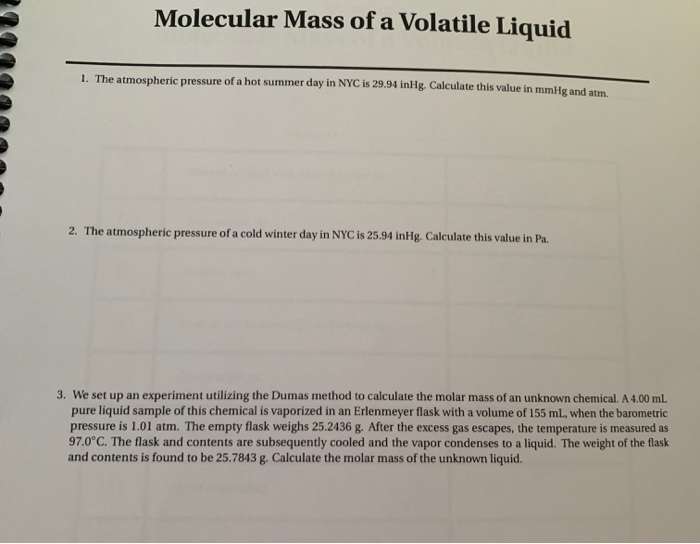Molecular Mass of a Volatile Liquid 1. The atmospheric pressure of a hot summer day in NYC is 29.94 inHg. Calculate this value in mmile and am 2. The atmospheric pressure of a cold winter day in NYC is 25.94 inHg. Calculate this value in Pa. 3. We set up an experiment utilizing the Dumas method to calculate the molar mass of an unknown chemical. A 4.00 mL pure liquid sample of this chemical is vaporized in an Erlenmeyer flask...

• ### i dont understand why the molecular weight i calculated (42.012 g/mol) for my unknown liquid (acetone)...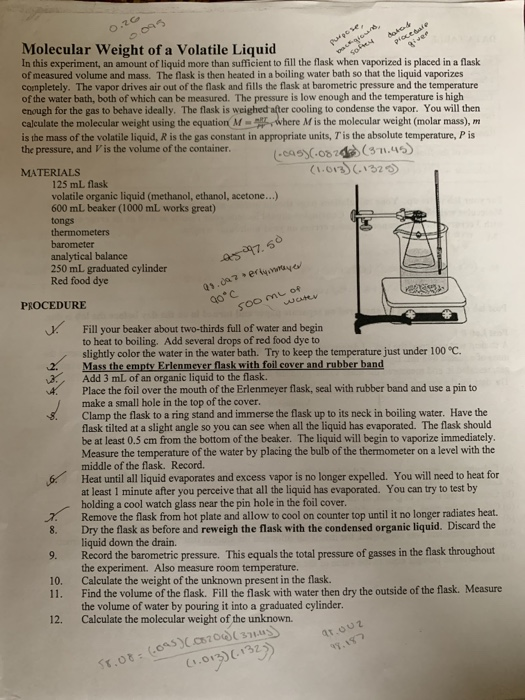i dont understand why the molecular weight i calculated (42.012 g/mol) for my unknown liquid (acetone) is less than the actual molecular weight (58.08 g/mol). what errors could i have made in my lab that would account for the difference. Molecular Weight of a Volatile Liquid In this experiment, an amount of liquid more than sufficient to fill the flask when vaporized is placed in a flask of measured volume and mass. The flask is then heated in a boiling...

• ### Prepare For It! Prelab Question A student weighs a flask, foil cover, and rubber band and...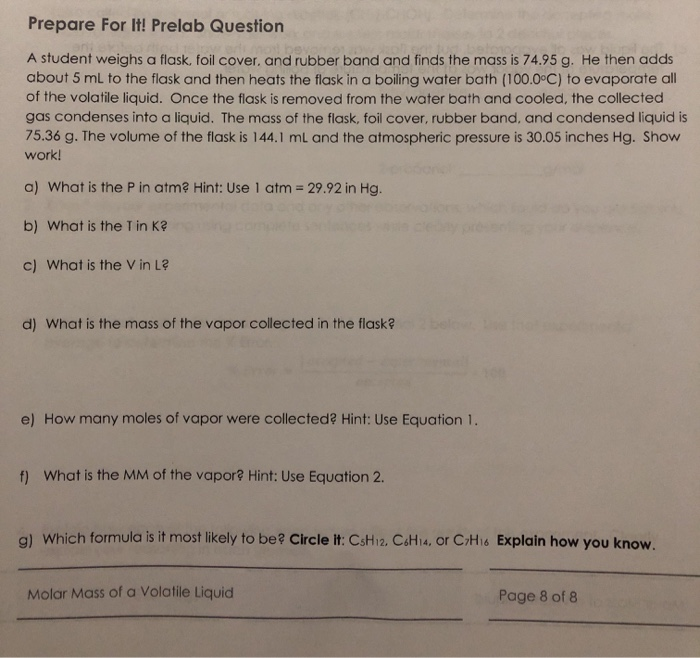Prepare For It! Prelab Question A student weighs a flask, foil cover, and rubber band and finds the mass is 74.95 g. He then adds about 5 mL to the flask and then heats the flask in a boiling water bath (100.0°C) to evaporate all of the volatile liquid. Once the flask is removed from the water bath and cooled, the collected gas condenses into a liquid. The mass of the flask, foil cover, rubber band, and condensed liquid is...

• ### A 0.704-g sample of a colorless liquid was vaporized in an evacuated 250.-mL flask at 121°C...

A 0.704-g sample of a colorless liquid was vaporized in an evacuated 250.-mL flask at 121°C to give a pressure of 786 mmHg. What is the molecular weight of this substance? Molecular weight = amu

Free Homework App